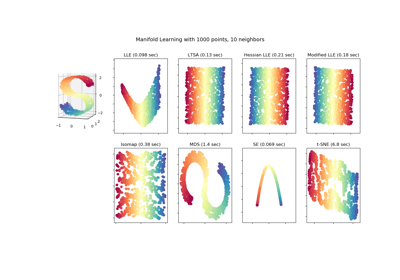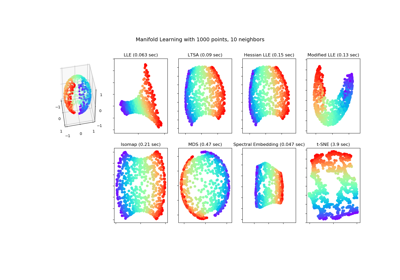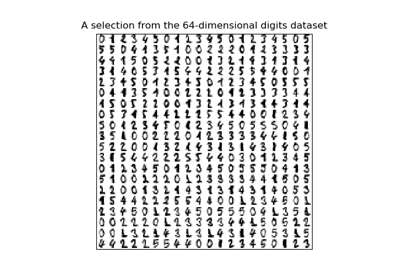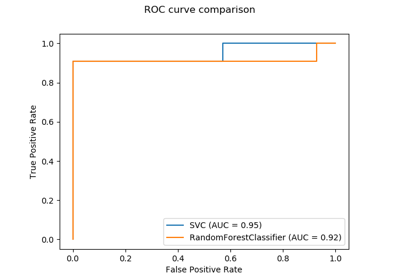# sklearn.manifold.Isomap¶

class sklearn.manifold.Isomap(n_neighbors=5, n_components=2, eigen_solver='auto', tol=0, max_iter=None, path_method='auto', neighbors_algorithm='auto', n_jobs=None, metric='minkowski', p=2, metric_params=None)[source]

Isomap Embedding

Non-linear dimensionality reduction through Isometric Mapping

Read more in the User Guide.

Parameters
n_neighborsinteger

number of neighbors to consider for each point.

n_componentsinteger

number of coordinates for the manifold

eigen_solver[‘auto’|’arpack’|’dense’]

‘auto’ : Attempt to choose the most efficient solver for the given problem.

‘arpack’ : Use Arnoldi decomposition to find the eigenvalues and eigenvectors.

‘dense’ : Use a direct solver (i.e. LAPACK) for the eigenvalue decomposition.

tolfloat

Convergence tolerance passed to arpack or lobpcg. not used if eigen_solver == ‘dense’.

max_iterinteger

Maximum number of iterations for the arpack solver. not used if eigen_solver == ‘dense’.

path_methodstring [‘auto’|’FW’|’D’]

Method to use in finding shortest path.

‘auto’ : attempt to choose the best algorithm automatically.

‘FW’ : Floyd-Warshall algorithm.

‘D’ : Dijkstra’s algorithm.

neighbors_algorithmstring [‘auto’|’brute’|’kd_tree’|’ball_tree’]

Algorithm to use for nearest neighbors search, passed to neighbors.NearestNeighbors instance.

n_jobsint or None, default=None

The number of parallel jobs to run. None means 1 unless in a joblib.parallel_backend context. -1 means using all processors. See Glossary for more details.

metricstring, or callable, default=”minkowski”

The metric to use when calculating distance between instances in a feature array. If metric is a string or callable, it must be one of the options allowed by sklearn.metrics.pairwise_distances for its metric parameter. If metric is “precomputed”, X is assumed to be a distance matrix and must be square. X may be a Glossary.

New in version 0.22.

pint, default=2

Parameter for the Minkowski metric from sklearn.metrics.pairwise.pairwise_distances. When p = 1, this is equivalent to using manhattan_distance (l1), and euclidean_distance (l2) for p = 2. For arbitrary p, minkowski_distance (l_p) is used.

New in version 0.22.

metric_paramsdict, default=None

Additional keyword arguments for the metric function.

New in version 0.22.

Attributes
embedding_array-like, shape (n_samples, n_components)

Stores the embedding vectors.

kernel_pca_object

KernelPCA object used to implement the embedding.

nbrs_sklearn.neighbors.NearestNeighbors instance

Stores nearest neighbors instance, including BallTree or KDtree if applicable.

dist_matrix_array-like, shape (n_samples, n_samples)

Stores the geodesic distance matrix of training data.

References

R7f4d308f5054-1

Tenenbaum, J.B.; De Silva, V.; & Langford, J.C. A global geometric framework for nonlinear dimensionality reduction. Science 290 (5500)

Examples

>>> from sklearn.datasets import load_digits
>>> from sklearn.manifold import Isomap
>>> X.shape
(1797, 64)
>>> embedding = Isomap(n_components=2)
>>> X_transformed = embedding.fit_transform(X[:100])
>>> X_transformed.shape
(100, 2)


Methods

 fit(self, X[, y]) Compute the embedding vectors for data X fit_transform(self, X[, y]) Fit the model from data in X and transform X. get_params(self[, deep]) Get parameters for this estimator. Compute the reconstruction error for the embedding. set_params(self, \*\*params) Set the parameters of this estimator. transform(self, X) Transform X.
__init__(self, n_neighbors=5, n_components=2, eigen_solver='auto', tol=0, max_iter=None, path_method='auto', neighbors_algorithm='auto', n_jobs=None, metric='minkowski', p=2, metric_params=None)[source]

Initialize self. See help(type(self)) for accurate signature.

fit(self, X, y=None)[source]

Compute the embedding vectors for data X

Parameters
X{array-like, sparse graph, BallTree, KDTree, NearestNeighbors}

Sample data, shape = (n_samples, n_features), in the form of a numpy array, sparse graph, precomputed tree, or NearestNeighbors object.

yIgnored
Returns
selfreturns an instance of self.
fit_transform(self, X, y=None)[source]

Fit the model from data in X and transform X.

Parameters
X{array-like, sparse graph, BallTree, KDTree}

Training vector, where n_samples in the number of samples and n_features is the number of features.

yIgnored
Returns
X_newarray-like, shape (n_samples, n_components)
get_params(self, deep=True)[source]

Get parameters for this estimator.

Parameters
deepbool, default=True

If True, will return the parameters for this estimator and contained subobjects that are estimators.

Returns
paramsmapping of string to any

Parameter names mapped to their values.

reconstruction_error(self)[source]

Compute the reconstruction error for the embedding.

Returns
reconstruction_errorfloat

Notes

The cost function of an isomap embedding is

E = frobenius_norm[K(D) - K(D_fit)] / n_samples

Where D is the matrix of distances for the input data X, D_fit is the matrix of distances for the output embedding X_fit, and K is the isomap kernel:

K(D) = -0.5 * (I - 1/n_samples) * D^2 * (I - 1/n_samples)

set_params(self, **params)[source]

Set the parameters of this estimator.

The method works on simple estimators as well as on nested objects (such as pipelines). The latter have parameters of the form <component>__<parameter> so that it’s possible to update each component of a nested object.

Parameters
**paramsdict

Estimator parameters.

Returns
selfobject

Estimator instance.

transform(self, X)[source]

Transform X.

This is implemented by linking the points X into the graph of geodesic distances of the training data. First the n_neighbors nearest neighbors of X are found in the training data, and from these the shortest geodesic distances from each point in X to each point in the training data are computed in order to construct the kernel. The embedding of X is the projection of this kernel onto the embedding vectors of the training set.

Parameters
Xarray-like, shape (n_queries, n_features)

If neighbors_algorithm=’precomputed’, X is assumed to be a distance matrix or a sparse graph of shape (n_queries, n_samples_fit).

Returns
X_newarray-like, shape (n_queries, n_components)

## Examples using sklearn.manifold.Isomap¶